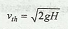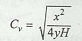### Hydraulic Coefficients

Hydraulic Coefficients include Coefficient of contraction, Coefficient of velocity, Coefficient of discharge and Coefficient of resistance. The following are the hydraulic coefficients:

1. Coefficient of contraction (Cc). It is defined as the ratio of area of jet at *vena contracta (ac) to the area of orifice (a).

The point at which the streamlines first become parallel is called vena contracta. The cross-sectional area of the jet at vena contrata is less than that of the orifice. The theoretical velocity of jet at vena contracta is given byThis expression is called Torricelli's theorem.

2. Coefficient of velocity (Cv). It is defined as the ratio of the actual velocity of the jet at vena contracta (v) to the theoretical velocity.

3. Coefficient of discharge (Cd). It is defined as the ratio of the actual discharge through the orifice (Q) to the theoretical discharge (Qth). The coefficient of discharge is equal to the product of Cc and Cv.

4. Coefficient of resistance (Cr). It is defined as the ratio of loss of head in the orifice to the head of water available at the exit of the orifice.

Note: The coefficient of velocity is determined experimentally by using the following relation, i.e.where
x = Horizontal distance,
y = Vertical distance, and